## NCERT Solutions for Class 12 Physics Chapter 10 Wave Optics

NCERT Solutions for Class 12 Physics Chapter 10 Wave Optics are part of NCERT Solutions for Class 12 Physics. Here we have given. NCERT Solutions for Class 12 Physics Chapter 10 Wave Optics

 Board CBSE Textbook NCERT Class Class 12 Subject Physics Chapter Chapter 10 Chapter Name Wave Optics Number of Questions Solved 21 Category NCERT Solutions

Question 1.
Monochromatic light of wavelength 589 nm is incident from air on a water surface. What are the wavelength, frequency and speed of
(a) reflected, and
(b) refracted light ? Refractive index of water is 1.33. (C.B.S.E. Sample Paper 1991)
(a) For reflected light wavelength is unchanged i. e.
X = 589 x 10-9 m = 589 nm
Also, speed of light in air c = 3 x 108 m s -1Question 2.
What is the shape of the wavefront in each of the following cases:
(a) Light diverging from a point source.
(b) Light emerging out of a convex lens when a point source is placed at its focus.
(c) The portion of the wavefront of light from a distant star intercepted by the Earth.
(a) Spherical shape
(b) Plane wavefront
(c) Plane wavefront

Question 3.
(a) The refractive index of glass is 1.5. What is the speed of light in glass? (Speed of light in a vacuum is 3.0 x 108 m s-1)
(b) Is the speed of light in glass independent of the colour of light? If not, which of the two colours red and violet travels slower in a glass prism ?
(a)
Here ,n=105,c=3.0 x 108 ms-1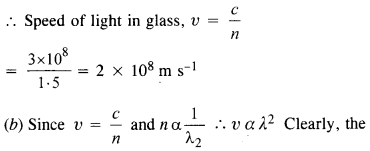speed of light when passing through glass depends on colour of light. λr > λυ , therefore the speed of violet light is less than the red light.

Question 4.
In a Young’s double-slit experiment, the slits are separated by 0.28 mm and the screen is placed 1.4 m away. The distance between the central bright fringe and the fourth bright fringe is measured to be 1.2 Determine the wavelength of light used in the experiment.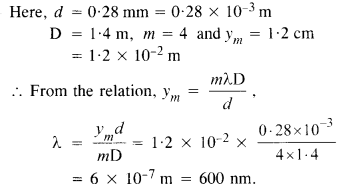Question 5.
In Young’s double-slit experiment using monochromatic light of wavelength λ, the intensity of light at a point on the screen where path difference is λ is K units. What is the intensity of light at a point where path difference is λ/2 ?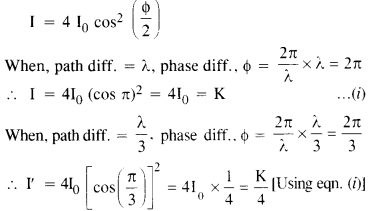Question 6.
A beam of light consisting of two wavelengths 650 nm and 520 nm is used to obtain interference fringes in a Young’s double-slit experiment.
(a) Find the distance of the third bright fringe on the screen from the central maximum for wavelength 650 nm.
(b) What is the least distance from the central maximum where the bright fringes due to both the wavelengths coincide ?
The distance between two slits is 2 mm and distance between the plane of the slits and the screen is 1.2 m.
(a) λ = 650 nm = 650 x 10-9 m,
d = 2 mm = 2 x 10-3 m,
D = 1.2 m
Distance of mth bright fringe from the central maximum is given by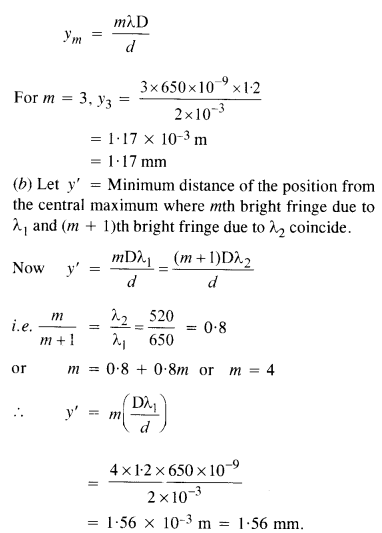Question 7.
In a double-slit experiment, the angular width of a fringe is found to be 0.2° on a screen placed 1 m away. The wavelength of light used is 600 nm. What will be the angular width of the fringe if the entire experiment apparatus is immersed in water ? Take
refractive index of water to be $$\frac { 4 }{ 3 }$$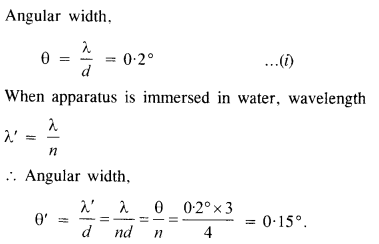Question 8.

What is the Brewster angle for air to glass transition? (Refractive index of glass = 1.5.)
µ = 1.5
According to Brewster’s law,
µ = tan p
tan p = 1.5
⇒ p = 56.31º

Question 9.
Light of wavelength 5000 A falls on a plane reflecting surface. What are the wavelength and frequency of the reflected light ? For what angle of incidence is the reflected ray normal to the incident ray ?
Here X = 5000 A = 5000 X 10-10 m,
c =3 x 108 m s-1
Wave length of reflected light
= Wavelength of incident light = 5000 ÅQuestion 10.

Estimate the distance for which ray optics is good approximation  for an aperture  of
4 mm and wavelength 400 nm.
Here X = 400 nm = 400 x 10-9 m, Aperture, a = 4 mm = 4 x 10-3 m
.’. Distance for which  ray optics is a good approximation is Fresnel’s distance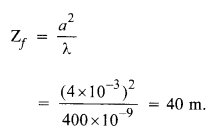Question 11.

The 6563 Å Hα line emitted by hydrogen in a star is found to be red-shifted by 15Å. Estimate the speed with which the star is receding from the Earth.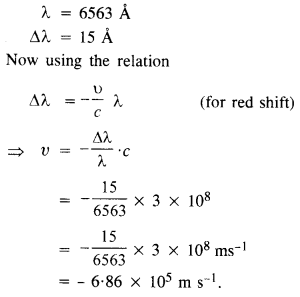Negative sign shows that the star is receding away from the earth.

Question 12.
Explain how Corpuscular theory predicts the speed of light in a medium, say, water, to be greater than the speed of light in vacuum. Is the prediction confirmed by experimental determination of the speed of light in water ? If not, which alternative picture of light is consistent with experiment ?
According to Corpuscular theory, when light in the form of particles enters into denser medium from a rarer medium, a force of attraction comes into play on the particles normal to the surface. Thus, the component of velocity normal to the surface of water increases whereas the component of velocity parallel to surface does not change. Therefore,velocity in water is greater than velocity of light in air. However, in actual case, c > υ. Huygen’s wave theory of light is consistent with the experiment.

Question 13.
You have learnt in the text how Huygens’ principle leads to the laws of reflection and refraction. Use the same principle to deduce directly that a point object placed in front of a plane mirror produces a virtual image whose distance from the mirror is equal to the object distance from the mirror.
Let there be a point object A at a distance y from a plane mirror. Treating this point to be a point source of light, we can assume spherical wavefronts progressing from A of radius y. Let there be no mirror then after time t, the wavefront will reach A’ as wavefront I. If a mirror is placed as shown in the figure then image will be formed at A’ represented by II.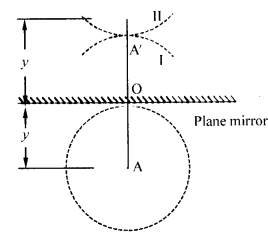It is seen that OA’ = OA i. e. virtual image is formed at a distance equal to the distance of object from the mirror.

Question 14.
Let us list some of the factors, which could possibly influence the speed of wave propagation:
(1) nature of the source,
(2) direction of propagation.
(3) the motion of the source and/or observer.
(4) wavelength
(5) intensity of the wave.
On which of these factors, if any, does
(a) the speed of light in vacuum,
(b) the speed of light in a medium (say, glass or water), depend ?
(a) Speed of light in a vacuum is an absolute constant (universal constant). It is independent of any factor. It is independent of the relative motion between source and observer even.
(b)

1. Speed of light in a medium depends upon wavelength.
2. It is independent of the nature of the source and motion of the source relative to the medium.
3. It depends upon the properties of the medium of propagation and motion of the observer relative to the medium,
4. It is independent of the direction of propagation for isotropic medium,
5. It is independent of the intensity of the wave.

Question 15.
For sound waves, the Doppler formula for frequency shift differs slightly between the two situations :
(1) a source at rest; observer moving, and
(2) source moving ; observer at rest. The exact Doppler formulae for the case of light waves in vacuum are, however, strictly identical for these situations. Explain why this should be so. Would you expect the formulae to be strictly identical for the two situations in case of light travelling in a medium ?
Sound requires material medium for propagation. Though situations
(1) and (2) may correspond to the same relative motion, yet they are not identical physically as the motion of observer relative to medium may be different in both situations. Hence, Doppler effect for sound cannot be same in both situations. Light when passing through material medium is also governed by different Doppler formulae for
(1) a source at rest; observer moving and
(2) source moving; observer at rest.
But when light passes through vacuum the formulae become exactly same for the two different situations because speed of light and frequency/wavelength of light remain unchanged in vacuum.

Question 16.
In a double-slit experiment using light of wavelength 600 nm, the angular width of a fringe formed on a distant screen is 0.1°. What is the spacing between two slits?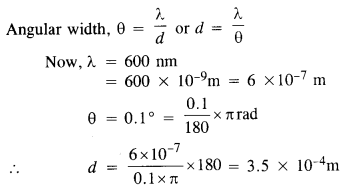Question 17.
(a)
In a single-slit diffraction experiment, the width of the slit is made double the original width. How does this affect the size and intensity of the central diffraction
band ?
(b) In what way is diffraction from each slit related to the interference pattern in a double-slit experiment? (C.B.S.E. 2013, 2013 )
(c)
When a tiny circular obstacle is placed in the path of light from a distant source, a bright spot is seen at the center of the shadow of the obstacle.
Explain why? (C. B. S. E. 2013 )
(d)
Two students are separated by a 7 m partition wall in a room 10 m high. If both light and sound waves can bend around obstacles, how is it that the students are unable to see each other even though they can converse easily ?   (C.B.S.E. 1990 )
(e)
Ray optics is based on the assumption that light travels in a straight line. Diffraction effects (observed when light propagates through small apertures/slits or around small obstacles) disprove this assumption. Yet the ray optics assumption is so commonly used in understanding location and several other properties of images in optical instruments. What is the justification?   (C.B.S.E. 1990)
(a) The width of central maxima = 2λD/d.
When the width (d) of slit is doubled, then the width of central diffraction maxima reduces to half and the intensity of the central band increases four times as amplitude of light wave is doubled.
(b) Intensity of fringes produced in the double-slit experiment is changed due to diffraction pattern superposing due to each slit.
(c) Light waves diffract at the edges of the circular obstacle. These diffracted waves interfere constructively and give rise to the bright spot at the center of the geometrical shadow.
(d) Diffraction is observed when the wavelength of the wave is of the order of the size of the obstacle. The wavelength of sound wave (≈ 0.33 m) is larger than the light wave (≈10-7 m) and is also comparable to wall, so diffraction of sound waves takes place and hence the students can converse easily. On the other hand, the wavelength of light is very small as compared to the obstacle e. 1 m high wall so the diffraction of light waves does not take place.
(e) In optical instruments, size of apertures are much larger than the wavelength of light. So diffraction of light is negligible. Hence, the assumption that light can travel in straight line is used in optical instruments.

Question 18.
Two towers on top of two hills are 40 km apart. The line joining them passes 50 m above a hill halfway between the towers. What is the longest wavelength of radio waves, which can be sent between the towers without appreciable diffraction effects ?
If A and B are two hills and C is the hill peak midwayQuestion 19.
A parallel beam of light of wavelength 500 nm falls on a narrow slit and the resulting diffraction pattern is observed on a screen 1 m away. It is observed that the first minimum is at a distance of 2-5 mm from the center of the screen. Find the width of the slit.( C.B.S.E. 2013)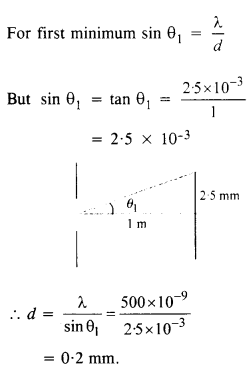Question 20.
(a) When a low flying aircraft passes overhead, we sometimes notice a slight shaking of the picture on our TY screen. Suggest a possible explanation.
(b) As you have learned in the text, the principle of linear superposition of wave displacement is basic to understanding distributions in diffraction and interference patterns. What is the justification of this principle?